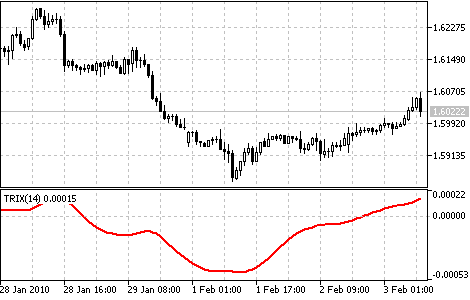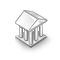Interesting script?
So post a link to it -
let others appraise it

You liked the script? Try it in the MetaTrader 5 terminal# Triple Exponential Average (TRIX) - indicator for MetaTrader 5

Views:
18293
Rating:
Published:
2010.02.03 14:04
Updated:
2016.11.22 07:32

Triple Exponential Average (TRIX) was developed by Jack Hutson as an oscillator of the overbought/oversold market conditions.

It can also be used as the Momentum indicator. Triple smoothing is used for removing the cyclic components in price movements with the period less than that of TRIX.

The zone is used as the indicator of overbought or oversold state (positive and negative respectively). The signal to by is crossing of the zero line from below, or "bulls'" divergence; the signal to sell is the indicator's crossing the zero line from above, or "bears'" divergence with prices. The distinctive feature of the indicator is the perfect filtration of price noises and absence of lag that is so typical of most moving averages.Triple Exponential Average Indicator

Calculation:

First the Exponential Moving Average of a price is calculated:
EMA1(i) = EMA(Price, N, i)
where:
• Price(i) - current price;
• N - EMA period;
• EMA1(i) - current value of the Exponential Moving Average.
Then the second smoothing of the obtained average is performed - double exponential smoothing:
EMA2(i) = EMA(EMA1, N, i).
The double Exponential Moving Average is smoothed exponentially once again - we get the Triple Exponential Moving Average:
EMA3(i) = EMA(EMA2, N, i);
Now the indicator itself is calculated:
TRIX(i) = (EMA3(i) - EMA3(i - 1))/ EMA3(i-1)Variable Index Dynamic Average (VIDYA)

This oscillator measures the ratio between the sum of positive increments and sum of negative increments for a certain period.Triple Exponential Moving Average (TEMA)

TEMA can be used instead of traditional moving averages. It can be used for smoothing price data, as well as for smoothing other indicators.MovingAverages

The MovingAverages library contains functions for calculation of different types of moving averages.LoongClock

A very simple sample of clock